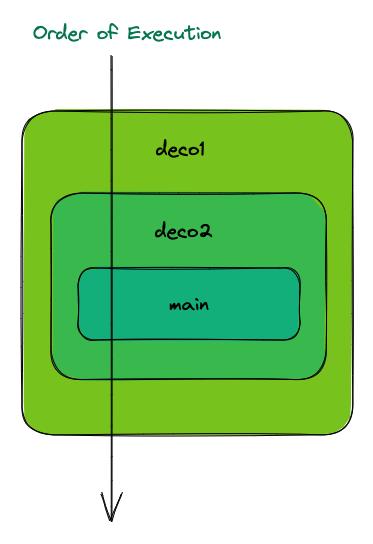# 看了肯定會的 Python 裝飾子(decorator)教學

Last updated on  Mar 10, 2023  in  Python 程式設計 - 中階  by  Amo Chen  ‐ 6 min read

Python 的 decorator (或稱裝飾子)是一個非常有用的功能，它的重要程度可以說是沒用過或不會用 decorator 的人就等於沒學過 Python, 甚至在一些常見的框架(Framework)，例如 Flask, FastAPI, Django 都提供各種方便的 decorator 供大家使用。

``````@debug
@cache
def sum(a, b):
return a + b
``````

• Python 3

### 開始之前 #

``````def sum(a, b):
print('a =', a)
print('b =', b)
print('a + b =', a + b)
return a + b
``````

``````def sum(a, b):
return a + b

def debug(func):
print('接到 func', func.__name__)
# 我想在這裡 debug
return func

debug_sum = debug(sum)
debug_sum(1, 2)
``````

p.s. 每個 function 都有 1 個屬性 `__name__` 可以取得函式名稱

``````接到 func sum
``````

#### 怎麼解決攔截傳入參數的部分？ #

``````def sum(a, b):
return a + b

def debug(func):
print('接到 func', func.__name__)
def wrapper(*args, **kwargs):
print('幫忙代入 args', args)
print('幫忙代入 kwargs', kwargs)
func(*args, **kwargs)
return wrapper

debug_sum = debug(sum)
debug_sum(1, 2)
print('debug_sum', debug_sum)
``````

``````接到 func sum

debug_sum <function debug.<locals>.wrapper at 0x107966430>
``````

``````debug(sum)(1, 2)
``````

``````def sum(a, b):
return a + b

def debug(func):
print('接到 func', func.__name__)
def wrapper(*args, **kwargs):
print('幫忙代入 args', args)
print('幫忙代入 kwargs', kwargs)
result = func(*args, **kwargs)
print(func.__name__, '執行結果', result)
return wrapper

debug_sum = debug(sum)
debug_sum(1, 2)
``````

``````接到 func sum

sum 執行結果 3
``````

``````def debug(func):
print('接到 func', func.__name__)
def wrapper(*args, **kwargs):
print('幫忙代入 args', args)
print('幫忙代入 kwargs', kwargs)
result = func(*args, **kwargs)
print(func.__name__, '執行結果', result)
return wrapper

debug_print = debug(print)
debug_print(1, 2, '3')
``````

### `@` 語法糖(Syntactic sugar) #

``````@debug
def sum(a, b):
return a + b
``````

``````import time

def timeit(func):
def wrapper():
s = time.time()
func()
print(func.__name__, 'total time', time.time() - s)
return wrapper

@timeit
def sleep_10s():
time.sleep(10)

sleep_10s()
``````

``````sleep_10s total time 10.000677108764648
``````

### 多個裝飾子的執行順序 #

``````@timeit
@api
@auth_required
@cache
def get_profile():
...(略)
``````

``````def deco1(func):
def wrapper():
print('deco1')
func()
print('deco1 end')
return wrapper

def deco2(func):
def wrapper():
print('deco2')
func()
print('deco2 end')
return wrapper

@deco1
@deco2
def main():
print('main')

main()
``````

``````deco1
deco2
main
deco2 end
deco1 end
``````### functools.wraps #

``````import time

def timeit(func):
def wrapper():
s = time.time()
func()
print(func.__name__, 'total time', time.time() - s)
return wrapper

@timeit
def sleep_10s():
"""sleep 10s"""
time.sleep(10)

print('func', sleep_10s.__name__)
print('doc', sleep_10s.__doc__)
``````

``````func wrapper
doc string None
``````

``````import time

from functools import wraps

def timeit(func):
@wraps(func)
def wrapper():
s = time.time()
func()
print(func.__name__, 'total time', time.time() - s)
return wrapper

@timeit
def sleep_10s():
"""sleep 10s"""
time.sleep(10)

print('func', sleep_10s.__name__)
print('doc', sleep_10s.__doc__)
``````

``````func sleep_10s
doc string sleep 10s
``````

### 類別裝飾子(Class-based decorators) #

``````class ClsDeco:
def __init__(self, func):
self.func = func

def __call__(self):
print("before calling", self.func.__name__)
self.func()
print("after calling", self.func.__name__)

@ClsDeco
def say_hello():
print("Hello!")

say_hello()
``````

``````before calling say_hello
Hello!
after calling say_hello
``````

``````class ClsDeco:
def __init__(self, func):
self.func = func

def __call__(self):
print("before calling", self.func.__name__)
self.func()
print("after calling", self.func.__name__)

def say_hello():
print("Hello!")

ClsDeco(say_hello)()
``````

### 更複雜的 decorator - 可設定參數的裝飾子 #

``````@retry(max=3)
def get_stock_price():
pass
``````

``````r = retry(max=3)

@r
def get_stock_price():
pass
``````

``````def retry(max=1):
class Wrapper:
def __init__(self, func):
self.func = func

def __call__(self):
retried = 0
while retried < max:
try:
self.func()
except Exception:
retried += 1
print('Failed. Going to try again (', retried, ')')
else:
break
return Wrapper

@retry(max=3)
def get_stock_price():
raise ValueError

get_stock_price()
``````

``````Failed. Going to try again ( 1 )
Failed. Going to try again ( 2 )
Failed. Going to try again ( 3 )
``````

### 總結 #

Decorator 是一個非常實用的模式/功能，它可以讓我們輕鬆地在既有基礎上疊加額外的功能，除了使程式碼更加簡潔、易讀之外，還可以增加複用性。

https://peps.python.org/pep-0318/